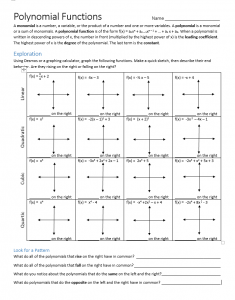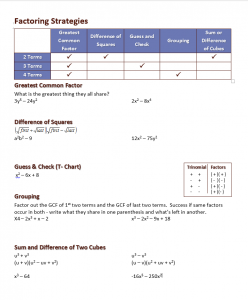# Polynomial Functions

//Polynomial Functions

## Graphing Polynomial Functions

A monomial is a number, a variable, or the product of a number and one or more variables. A polynomial is a monomial or a sum of monomials. A polynomial function is of the form f(x) = anxn + an – 1xn – 1 + … + a­1 x + a. When a polynomial is written in descending powers of x, the number in front (multiplied by the highest power of x) is the leading coefficient. The highest power of x is the degree of the polynomial. The last term is the constant. Often the best way to learn about functions is to discover their characteristics. Use the Polynomial Functions Exploration and Desmos or a graphing calculator to learn about end behaviors of these functions.Polynomial Functions Lesson 4-1 Notes

## Adding, Subtracting, and Multiplying Polynomials

Alg II Lesson 4-2 worksheet

Adding, Subtracting, and Multiplying Polynomials Lesson 4-2 notes

## Dividing Polynomials

### Synthetic Division

Synthetic Division Practice

### Polynomial Challenge

Understand the connection between factors, zeros, and the polynomials.

Review Lessons 4-1 – 4-3

## Factoring Polynomials

Factoring-StrategiesLesson 4-4

## Fundamental Theorem of Algebra

By | 2017-11-13T22:02:00+00:00 October 21st, 2015|Algebra II|0 Comments

### About the Author: Stacie Bender